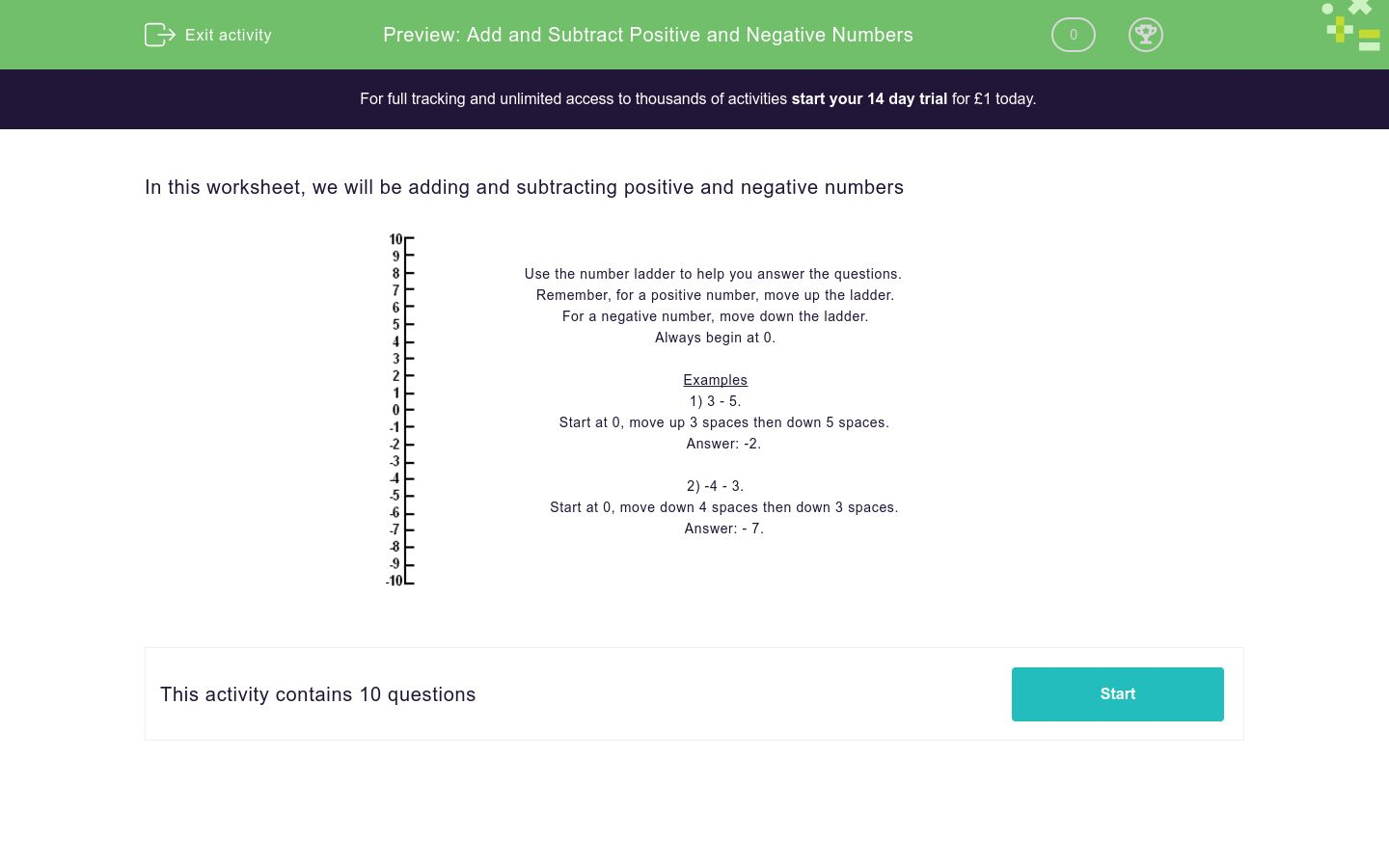# Add and Subtract Positive and Negative Numbers

In this worksheet, students carry out addition and subtraction of positive and negative numbers.Key stage:  KS 3

Curriculum topic:   Number

Curriculum subtopic:   Use Four Operations for All Numbers

Difficulty level:### QUESTION 1 of 10

In this worksheet, we will be adding and subtracting positive and negative numbersUse the number ladder to help you answer the questions. Remember, for a positive number, move up the ladder. For a negative number, move down the ladder. Always begin at 0. Examples 1) 3 - 5.     Start at 0, move up 3 spaces then down 5 spaces.     Answer: -2.      2) -4 - 3.     Start at 0, move down 4 spaces then down 3 spaces.     Answer: - 7.

Work out:

1 - 7 + 5 = ________

Work out:

2 - 3 + 4 = ________

Work out:

-7 - 2 = ________

Work out:

4 - 7 + 3 = ________

Work out:

-7 + 4 + 5 = ________

Work out:

-9 + 1 - 2 = ________

Work out:

-9 - 9 = ________

Work out:

7 - 1 - 10 = ________

Work out:

-2 + 8 + 6 - 5 =  ________

Work out:

50 - 50 -50 -50 = ________

• Question 1

Work out:

1 - 7 + 5 = ________

-1
• Question 2

Work out:

2 - 3 + 4 = ________

3
• Question 3

Work out:

-7 - 2 = ________

-9
• Question 4

Work out:

4 - 7 + 3 = ________

0
• Question 5

Work out:

-7 + 4 + 5 = ________

2
• Question 6

Work out:

-9 + 1 - 2 = ________

-10
• Question 7

Work out:

-9 - 9 = ________

-18
• Question 8

Work out:

7 - 1 - 10 = ________

-4
• Question 9

Work out:

-2 + 8 + 6 - 5 =  ________

7
• Question 10

Work out:

50 - 50 -50 -50 = ________

-100
---- OR ----

Sign up for a £1 trial so you can track and measure your child's progress on this activity.

### What is EdPlace?

We're your National Curriculum aligned online education content provider helping each child succeed in English, maths and science from year 1 to GCSE. With an EdPlace account you’ll be able to track and measure progress, helping each child achieve their best. We build confidence and attainment by personalising each child’s learning at a level that suits them.

Get started